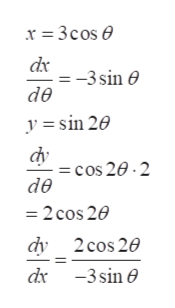# Find the points on the curve where the tangent is horizontal or vertical. If you have a graphing device, graph the curve to check your work.x = 3cos(θ)y = sin(2θ)

Question

Find the points on the curve where the tangent is horizontal or vertical. If you have a graphing device, graph the curve to check your work.
x = 3cos(θ)
y = sin(2θ)

check_circle

Step 1

Refer to the question, we have to find the points on the curve where the tangent is horizontal or vertical.

Step 2

Use the following formula for the derivative of sinx and cos x as,

Step 3

Use differentiation to get the tang...help_outlineImage Transcriptionclose-3 sin de y=sin 20 =cos 20-2 de =2 cos 20 dy 2cos 20 d 3sin fullscreen

### Want to see the full answer?

See Solution

#### Want to see this answer and more?

Solutions are written by subject experts who are available 24/7. Questions are typically answered within 1 hour.*

See Solution
*Response times may vary by subject and question.
Tagged in

### Derivative